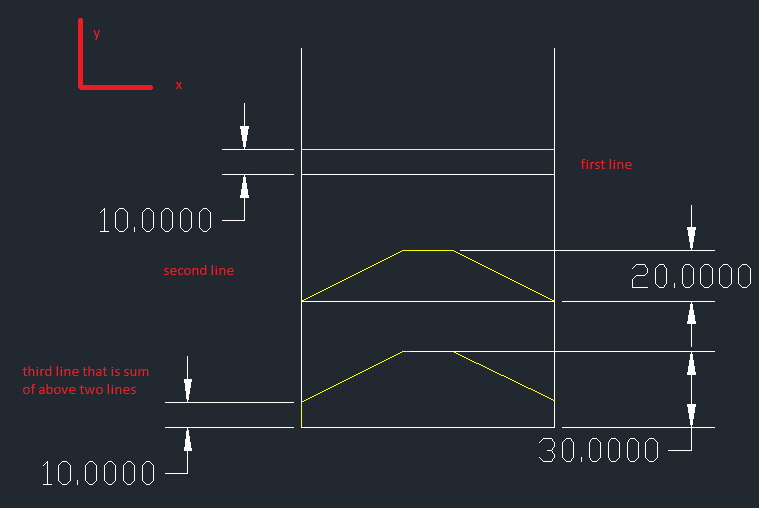## Recommended PostsI am quite the novice with Autocad and do not know how to approach this problem.

I have 2 different lines defined with x and y coordinates.

I want a third line that uses the common x coordinate and a y coordinate that is the sum of the two coordinates.

If this is possible, can i define which mathematical function i use so that i can add/subtract or even use a formula to define?

Thanks very much for your timeFurther Notes:

The example above is very simple. I have 2 complex curves that go into positive and negative values of y (speaking from a mathematical perspective). I need an easy way to intersect the complex curves at a specified interval and add them at each of those intervals.

For example i may a sine function going inbetween pi and -pi over a distance equal to 2. I then need to split the curves in intervals of 0.1 and add them together.## Join the conversation

You can post now and register later. If you have an account, sign in now to post with your account.
Note: Your post will require moderator approval before it will be visible.×   Pasted as rich text.   Restore formatting

Only 75 emoji are allowed.